# Preparation Manual

## Section 4: Sample Selected-Response Questions TX PACT: Physics: Grades 7–12 (739)

This section presents some sample exam questions for you to review as part of your preparation for the exam. To demonstrate how each competency may be assessed, sample questions are accompanied by the competency that they measure. While studying, you may wish to read the competency before and after you consider each sample question. Please note that the competency statements do not appear on the actual exam.

The correct answer is provided for each sample exam question. The sample questions are included to illustrate the formats and types of questions you will see on the exam; however, your performance on the sample questions should not be viewed as a predictor of your performance on the actual exam.

The following reference materials will be available to you during the exam:

### Domain I—Nature of Science

#### Competency 001—Understand principles and procedures of scientific inquiry.

Data for the variables x and y are collected during an experiment and given in the table below.

 x y 5 10 15 20 25 30 0.05 0.19 0.32 0.47 0.61 0.75

1. Which of the following best describes the relationship between x and y?

1. constant
2. linear
3. inverse
Option B is correct. This question requires the examinee to analyze scientific data. The x-values of the data in the table increase in increments of 5, that is, Delta x equals 5. The y-values increase in increments of 0.14, 0.13, 0.15, 0.14, and 0.14, respectively. Hence, the ratiodelta y over delta x is approximately constant and the relationship is best described as being linear.

#### Competency 002—Understand the history of science, its connections with other sciences, and the relationships among science, technology, and society.

2. Which of the following best describes how the invention of X-ray diffraction in physics contributed to the biological and chemical sciences?

1. by generating clear images of bacteria and viruses
2. by determining the structure of molecules
3. by discovering missing elements in the periodic table
4. by producing disease-resistant plant varieties
Option B is correct. This question requires the examinee to demonstrate knowledge of how science and technology affect each other. Because the wavelength of X-rays is of the same order as the size of molecules, X-ray diffraction allows scientists to infer structural properties of substances, such as DNA, by analyzing the patterns produced by the waves as they are diffracted from the substance.

### Domain II—Mechanics

#### Competency 003—Understand motion in one and two dimensions.

3. A ball at rest on level ground is kicked into the air. The ball strikes the ground 4.00 s later at a horizontal distance of 40.0 m from where it was kicked. What is the magnitude of the initial velocity of the ball?

1. 10.0 m slash s
2. 19.6 m slash s
3. 22.0 m slash s
4. 40.5 m slash s
Option C is correct. This question requires the examinee to apply properties of vectors to analyze projectile motion. Since the ball travels a horizontal distance of 40.0 m in 4.00 s, the horizontal component of its initial velocity is 10.0 m slash s. The ball is in flight for a total of 4.00 s, so it reaches its maximum height in 2.00 s. Using the equation v left parenthesis t right parenthesis equals negative 9.8t plus v subscript 0 subscript y and v left parenthesis 2.00 right parenthesis equals 0.00 gives v subscript 0 subscript y equals 19.6 m slash s. The magnitude of the initial velocity is thereforev 0 equals square root left parenthesis 10.0 right parenthesis squared plus left parenthesis 19.6 right parenthesis squared equals 22.0 m slash s.

#### Competency 004—Understand forces as interactions and their effects on motion.

A rope with a tension of 10.0 N is attached to a 2.00 kg mass. The rope makes an angle of 30.0 degrees with the horizontal.4. What is the normal force on the mass?

1. 5.0 N
2. 9.8 N
3. 14.6 N
4. 19.6 N
Option C is correct. This question requires the examinee to solve problems involving force and motion. There are three forces acting on the mass in the y-direction: the gravitational force, the normal force, and the vertical component of the tension in the string. The normal force is perpendicular to the surface of the mass and in the positive y-direction. From Newton's second law, sigma-summation F equals ma applied in the y-direction, sigma-summation F subscript y equals 0 equals negative mg plis N plus T sine 30 degrees. Using g equals 9.80 m slash s squared, substituting the values given, and solving for the normal force gives N equals 14.6 N.

#### Competency 005—Understand the conservation of energy and linear momentum.

A mass initially at rest at a height of 6r slides down a frictionless track and around a circular loop of radius r.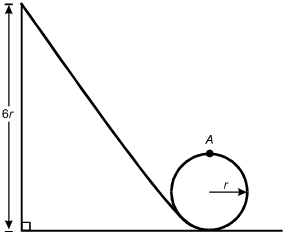5. What is the speed of the mass at the top of the circular loop (point A)?

1.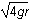The square root of 4gr
2.The square root of 6gr
3.The square root of 7gr
4.The square root of 8gr
Option D is correct. This question requires the examinee to apply the work-energy theorem to conservative systems. Since there are no frictional forces, the work done by friction is zero and the total mechanical energy is conserved. Therefore, mg left parenthesis 6r right parenthesis equals 1 half mv squared plus mg left parenthesis 2r right parenthesis. Solving this equation for v gives v equals the square root of 8gr.

#### Competency 006—Understand simple harmonic motion and rotational dynamics.

A mass on a spring is displaced from equilibrium and released. The graph below shows the velocity of the mass versus time.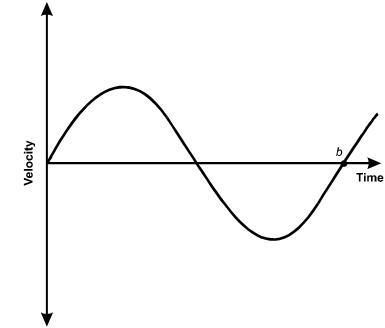A sinusoid line starts at the origin with an upward slope. After the upper curve and the lower curve, the line passes through the horizontal axis at point b.

6. Which statement about the mass at time b must be true?

1. It is located at its equilibrium position.
2. Its potential energy is equal to zero.
3. Its kinetic energy is at a maximum.
4. Its acceleration is at a maximum.
Option D is correct. This question requires the examinee to use graphs of trigonometric functions to analyze characteristics of simple harmonic motion. The graph shows the velocity of a mass oscillating on a spring. At point b the velocity is zero, so the mass is at one of its turning points and the displacement of the mass, x, is either A or negative A, where A is the amplitude of motion. Since the slope of the line tangent to the curve at b is positive, the acceleration is positive, so x equals negative A. Since F equals ma equals negative kx, the force, and therefore the acceleration, is maximum when x equals negative A.

### Domain III—Electricity and Magnetism

#### Competency 007—Understand properties of the electric field.

Three identical point charges are located on the vertices of an equilateral triangle of side length s.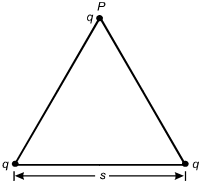7. What is the vertical component of the electrostatic force on the charge located on vertex P?

1. kq squared over s squared sine 30 degrees
2. kq squared over s squared cosine 30 degrees
3. 2kq squared over s squared sine 30 degrees
4. 2kq squared over s squared cosine 30 degrees
Option D is correct. This question requires the examinee to describe an electric force for a simple charge distribution. From Coulomb's law, the magnitude of the force on q from the left-most charge on the base of the triangle is F equals fraction kqq over s squared, and the component in the vertical direction is kqq over s squared cosine theta, where theta equals 30 degrees. This force points in the positive y-direction. The same applies for the force from the right-most charge. The net force is the superposition of the two forces, which is F equals >2kq squared over s squared cosine 30 degrees.

#### Competency 008—Understand properties of the magnetic field and electromagnetic induction.

A wire that carries conventional current I in the direction shown is in a magnetic field that points into the plane of the page.8. Which arrow shows the direction of the force on the wire?

1.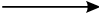2.3.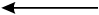4.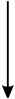Option C is correct. This question requires the examinee to analyze the magnetic force on a moving charge in a magnetic field. The diagram shows a magnetic field that points into the page. The wire carries conventional current, which implies positive charges moving toward the top of the page. Since start bold F end bold equals I samll l times start bold B end bold and small l represents the direction of the conventional current I, the right-hand rule can be used to cross start bold v end bold into start bold B end bold. This shows that the force on the wire points to the left, as shown in response C.

#### Competency 009—Understand properties of electric circuits.

9. A resistor is connected in series across the terminals of an ideal battery of voltage V. The resistor dissipates power W from the battery. A second, identical resistor is connected in series with the first resistor. What is the total power dissipated by the two resistors?

1. W over 2
2. W
3. 2W
4. 4W
Option A is correct. This question requires the examinee to analyze an electric circuit in terms of energy or power. The power dissipated by the resistor in the circuit is given by P equals IV equals W, where I is the current through the resistor. When a second resistor is added to the circuit, the effective resistance doubles. Since the voltage of the battery is the same, the current is 1 half I. Since there are two identical resistors in the circuit, the voltage across each is 1 half V and the power dissipated by each is P equals 1 fourth W. Therefore, the total power dissipated is P equals 1 half W.

### Domain IV—Waves

#### Competency 010—Understand the fundamental properties of waves.

10. A person standing 85 m from a wall shouts and hears the echo 0.50 s later. The person then plays a note of 440 Hz on a flute. What is the wavelength of the note in air?

1. 0.39 m
2. 0.77 m
3. 1.3 m
4. 2.6 m
Option B is correct. This question requires the examinee to solve a problem involving wave speed, frequency, and wavelength. Since the person hears the echo of the shout after 0.50 s, the speed of sound is 170 m slash 0.50 s or 340 m slash s. Since v equals f lambda, the wavelength is lambda equals 0.77 m.

#### Competency 011—Understand the characteristics of light and electromagnetic radiation.

11. A light-emitting diode (LED) on a consumer device emits 0.020 W of light of wavelength lambda equals 650 times 10 superscript negative 9 m. Which of the following expressions equals the number of photons emitted by the LED per second in terms of Planck's constant (h) and the speed of light (c)?

1. 0.020 lambda over hc

2. 0.020hcover lambda

3. 0.020h lambda over c

4. 0.020 over hc lambda
Option A is correct. This question requires the examinee to describe various phenomena using the photon model of light. The energy of a single photon is given by E equals hf. For light, c equals f lambda, so E equals hc over lambda;. Since the power of the LED is 0.020 W, dividing the power by the energy of a single photon gives the number of photons emitted per second.

### Domain V—Modern Physics

#### Competency 012—Understand thermal energy and the kinetic theory of matter.

A closed, insulated vessel consists of a paddle wheel immersed in 0.50 kg of water at room temperature. The shaft of the paddle wheel is attached to a motor that has an output of 25 W. The paddle wheel rotates, churning the water.12. Disregarding the heat capacity of the vessel and paddle, what is the change in temperature of the water if the motor is run for 5.0 minutes?

1. 0.03 degrees Celsius
2. 1.0 degrees Celsius
3. 1.8 degrees Celsius
4. 3.6 degrees Celsius
Option D is correct. This question requires the examinee to solve a problem related to specific heat and the first law of thermodynamics. The first law of thermodynamics states that Delta E equals Delta Q minus W, where W is the work done by the system. Since the vessel is insulated, Delta Q equals 0. Therefore, Delta E equals W, since work is done on the system as the paddle wheel churns the water. Therefore, c subscript w msubscript w Delta T equals left parenthesis 25 W right parenthesis left parenthesis 5 min right parenthesis left parenthesis 60 s slash min right parenthesis. Using the value c subscript w equals 4.19 times 10 cubed J slash left parenthesis kg dot degrees Celsius right parenthesis given in the Constants and Formulas and solving gives Delta T equals 3.6 degrees Celsius.

#### Competency 013—Understand fundamental ideas of modern physics.

Electrons from an electron gun pass through a double slit before striking a screen. A counter records the number of electrons that arrive at the screen as a function of x, the position along the screen. The distribution of the number of electrons arriving is shown below.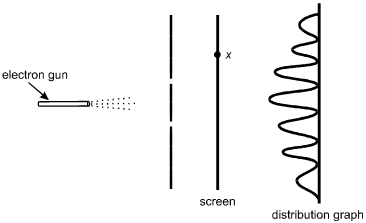The electron gun is on the left, pointed horizontally to the right. Dotted lines represent the electrons spraying toward the right. A vertical line with two breaks, located symmetrically above and below the level of the electron gun, is between the gun and a vertical line representing the screen. A point on the screen is labeled x, somewhat above the level of the upper slit. To the right of the screen is a distribution graph with an oscillating sinusoidal wave on a vertical baseline. The peaks are to the left of the baseline. The highest peak is in the center, at the level of the electron gun, with peaks progressively lower at the upper and lower ends of the graph.

13. According to the principles of quantum mechanics, the distribution pattern shown is a result of:

1. electrons interfering due to their wave properties.
2. electrons striking the edges of the slits while passing through.
3. photons interacting with each other on either side of the slits.
4. electrons interacting with photons prior to arriving at the screen.
Option A is correct. This question requires the examinee to demonstrate knowledge of the wave-particle duality. In the double-slit experiment shown, the distribution of the number of electrons arriving on the screen at various positions is a result of interference. Interference is a consequence of the wave properties of electrons.

#### Competency 014—Understand the fundamental principles of nuclear physics.

14. If superscript A over subscript Z base X represents an atomic nucleus prior to gamma decay, which of the following represents the nucleus after gamma decay?

1. superscript A over subscript Z base X

2. superscript A negative 1 over subscript Z base X

3. superscript A negative 1 over subscript Z base X

4. superscript A negative 4 over subscript Z negative 2 base X